Brodin, F. W., and Theliander, H. (2013). "High temperature TEMPO oxidation in a heterogeneous reaction system: An investigation of reaction kinetics, pulp properties, and disintegration behavior," BioRes. 8(4), 5925-5946.

#### Abstract

TEMPO oxidation was performed on never-dried bleached softwood kraft pulp fibres to study the influence of reaction temperature and the dosage of sodium hypochlorite in the oxidation treatment. It was found that oxidation at a high temperature shortened oxidation time, but it also resulted in more extensive degradation of the pulp. Harsh reaction conditions (high temperature and high dosage of sodium hypochlorite) enabled the rapid disintegration of pulp fibres with a low energy demand. The freeze-dried disintegrated pulp fibres had a high absorption capacity of saline liquid, 40 to 80 g/g, and retained 9 to 14 g saline liquid/g material in a standard centrifuge retention test. Four different models were investigated to describe the kinetics of TEMPO oxidation of pulp fibres. However, none of the models could adequately describe all the mechanisms involved in this reaction system. The results showed that the diffusion of hypochlorite ions was sufficiently fast compared to the chemical reactions. In contrast, the concentration of the catalytic compounds, NaBr and TEMPO, both had a strong influence on the reaction rate. Results show that the reactions have different phases, possibly with different rate-determining steps. It remains to be determined which reaction steps correspond to the different phases.

High Temperature TEMPO Oxidation in a Heterogeneous Reaction System: An Investigation of Reaction Kinetics, Pulp Properties, and Disintegration Behavior

Fredrik W. Brodin and Hans Theliander*

TEMPO oxidation was performed on never-dried bleached softwood kraft pulp fibres to study the influence of reaction temperature and the dosage of sodium hypochlorite in the oxidation treatment. It was found that oxidation at a high temperature shortened oxidation time, but it also resulted in more extensive degradation of the pulp. Harsh reaction conditions (high temperature and high dosage of sodium hypochlorite) enabled the rapid disintegration of pulp fibres with a low energy demand. The freeze-dried disintegrated pulp fibres had a high absorption capacity of saline liquid, 40 to 80 g/g, and retained 9 to 14 g saline liquid/g material in a standard centrifuge retention test. Four different models were investigated to describe the kinetics of TEMPO oxidation of pulp fibres. However, none of the models could adequately describe all the mechanisms involved in this reaction system. The results showed that the diffusion of hypochlorite ions was sufficiently fast compared to the chemical reactions. In contrast, the concentration of the catalytic compounds, NaBr and TEMPO, both had a strong influence on the reaction rate. Results show that the reactions have different phases, possibly with different rate-determining steps. It remains to be determined which reaction steps correspond to the different phases.

Keywords: Kinetics; Diffusion; TEMPO oxidation; Fibres; MFC; Absorption; Retention

Contact information: Forest Products and Chemical Engineering, Department of Chemical and Biological Engineering, Chalmers University of Technology, SE-412 96 Gothenburg, Sweden;

* Corresponding author: hanst@chalmers.se

INTRODUCTION

Cellulose is currently only used in a few different products, mostly various paper products, but the demand for new cellulose-based products, e.g., viscose and various cellulose derivatives, is growing. Because cellulose is the most abundant biomaterial on earth, it can be expected that cellulose-based products will find many new applications in the future, e.g., as replacement products in applications that, today, are dominated by products produced from fossil-based resources.

Cellulose extracted from wood using chemical pulping methods is an excellent starting material for developing new biomaterials. In many cases, however, it will be necessary to modify the cellulose properties to fulfill the needs of the new applications. There are many ways of modifying cellulose, and one of these is oxidizing the hydroxyl groups in the cellulose, thus forming new aldehyde and carboxylate groups. Primary hydroxyl groups can be oxidized selectively through so-called TEMPO oxidation, where a catalytic amount of 2,2,6,6-tetramethylpiperidine-1-oxyl (TEMPO) mediates the oxidation of cellulose and is regenerated by a primary oxidant (Bragd et al. 2004). This type of modification also causes a substantial decrease in the degree of polymerization (DP) (Saito and Isogai 2004), i.e., the molecular weight of the cellulose polymers decreases. Saito et al. (2006) found that TEMPO-oxidized pulp fibres could be disintegrated into microfibrillar cellulose (MFC) through treatment in a Waring blender. Furthermore, they reported that the disintegration of TEMPO-oxidized sulfite pulp was facilitated by a higher dosage of NaClO. Dang et al. (2007) reported that a greater amount of negatively charged carboxylate groups, after TEMPO oxidation, enhanced the water retention value (WRV), thus indicating enhanced fibre swelling. In contrast to this, Kitaoka et al. (1999) found that the WRV was almost unaffected, even though the amount of carboxylate groups was increased from 0.06 to 0.47 mmol/g.

Under alkaline conditions, TEMPO oxidation has been tested at low and moderate temperatures up to room temperature level. Isogai and Kato (1998) studied the influence of TEMPO oxidation conditions and found that higher temperature and longer oxidation time resulted in a lower DP in the oxidized pulps. Kitaoka et al. (1999) reported that the DP decreased rapidly when the pulp was subjected to TEMPO oxidation, but at a higher dosage of sodium hypochlorite charge, the DP leveled out at about 200. TEMPO oxidation can also be performed at near neutral conditions (Saito et al. 2009), using a TEMPO/NaClO/NaClO2 reaction system. The resulting pulp has almost the same DP as the starting pulp. However, there are also applications and processes where a high DP is a disadvantage or is of minor importance for the performance of the material, e.g., when the oxidized pulp is disintegrated and dried for usage in absorbent applications (Brodin and Theliander 2012). In such a material, there are other properties, such as the pore size distribution of the material and the available surface area, that have a greater influence on the absorption and retention properties of the material.

The kinetics of TEMPO oxidation has been studied in a homogeneous reaction system (TEMPO/NaClO) with methyl α-D glucopyranoside as the substrate (Bragd et al. 2000). The experiments in the kinetics study were conducted at 20 ˚C and with a 10% excess amount of hypochlorite ions relative to the amount of primary hydroxyl groups. It was found that the reaction could be described as a first-order reaction in TEMPO and the substrate. Furthermore, the authors suggested that the reaction between the nitrosium ion and the primary alcohol was the rate-determining step under the given experimental conditions. In an earlier study, de Nooy et al. (1995) found in experiments with TEMPO/NaBr/NaClO and methyl α-D glucopyranoside that the rate of carboxylate group formation was much faster (about seven times faster) than the rate of aldehyde group formation. TEMPO oxidation kinetics has also been studied for regenerated cellulose, e.g., rayon fibres (Sun et al. 2005), and for cotton linters (Dai et al. 2011), where the kinetics was modeled using a homogeneous reaction model. Sun et al. (2005) found that the experimental data could be well fitted to a first-order reaction expression with respect to the primary oxidant (NaClO). For cotton linters, Dai et al. (2011) found that the kinetics could be described by two first-order expressions, i.e., one for the rapid initial reaction and one for the remaining part of the reaction. These studies have been conducted with model compounds or with fibres; however, all of the studies assumed that mass-transfer resistance could be neglected. In TEMPO oxidation of fibrous substrates, the reactants must be transported from the liquid bulk to the reaction site, and dissolved reaction products must be transported from the reaction site in the fibre wall to the bulk liquid. This implies that mass transport phenomena, along with the geometry of the fibres, may influence the overall kinetics. To our knowledge, no study has approached the kinetics in TEMPO oxidation in a heterogeneous reaction system to investigate whether mass transfer has any impact on reaction rate.

The aim of this paper was twofold: The first aim was to obtain greater knowledge about how process conditions during TEMPO oxidation influence fibre disintegration into MFC, and the second aim was to determine how this influences the absorption properties of the foams obtained after freeze drying. The experimental data from reaction experiments were also used in the second part of the study to shed some light on the kinetics of TEMPO oxidation.

EXPERIMENTAL

Materials

The starting material used in this study was an industrial TCF bleached softwood kraft pulp produced from Scandinavian softwood. The pulp was never dried prior to the oxidation experiments, had 0.07 mmol negatively charged groups/g pulp, as determined by sorption of methylene blue, and had a DPV of 1250 estimated from intrinsic viscosity measurements (cf. “Methods”). The chemicals used in the TEMPO oxidation experiments were TEMPO (purity 98%, Sigma Aldrich), NaBr (purity 99.5%, Scharlau), and NaClO (10 to 15% available chlorine, Sigma Aldrich).

Methods

Oxidation

In the first part of this study, 12 oxidation experiments were conducted, and the two parameters that were varied were reaction temperature (25˚ C, 30˚ C, 40˚ C, and 50˚ C) and the dosage of sodium hypochlorite (2.5, 3.8, and 5.0 mmol NaClO/g pulp). The concentration of hypochlorite ions was determined by iodometric titration prior to the oxidation experiments. The oxidations were performed in a cylindrical glass vessel placed in a temperature-regulated water bath with a heater. First, 5.0 g o.d. of pulp was mixed with TEMPO (0.08 g), NaBr (0.5 g), and deionized water. To minimize the flocculation of the fibres, the pulp consistency in the reaction system was low (1%) and the suspension was continuously stirred both before and during the experiment. Before the oxidation was started, the temperature of the pulp suspension was heated to the reaction temperature. The reaction was initiated by adding the primary oxidant (NaClO), and the pH was maintained at 10.0 during the reaction using a pH-stat with 0.5 M sodium hydroxide. At the end of the addition of sodium hydroxide, the pulp was separated from the reaction liquid by filtration and washed with 2 L of deionized water, dewatered to about 15% pulp consistency, and stored at 4 ˚C.

The selectivity of forming carboxylate groups in the solid pulp material was calculated using Eq. .In the second part of the kinetic study, a 2-L baffled glass reaction vessel was used to obtain better mixing compared to a vessel without baffles. All other parts of the experimental setup remained unchanged. Oxidations were performed with variations in the concentration of the catalytic compounds, reaction temperature, and reaction pH. In the second part of the experiments, the concentration of hypochlorite ions in the reaction liquid was determined by iodiometric titration. Small samples of oxidation liquid, 1 to 2 mL per sample, were collected during the reaction and added to 15 mL of an acidic solution containing KI.

Disintegration and freeze drying

The pulps produced in the first set of oxidation experiments was also used in the disintegration and drying experiments. First, 2 g o.d. of pulp was disintegrated using an Ultra-Turrax T45/N (IKA) high-speed dispersing unit. The pulp consistency was 0.6% to obtain good mixing in all samples. The disintegration was conducted in a 500-mL plastic beaker. After 2 min (i.e., a total of 1 min of treatment per g of pulp), 80 g of wet material was removed (0.5 g of pulp) for later analyses. Then, the disintegration was continued for another 3.0 min (i.e., a total of 3 min of treatment per g of pulp). Both samples were put into plastic bottles and stored at 4 ˚C.

After disintegration, a part of the samples was freeze-dried to obtain porous absorbent materials. To do so, 20 g of wet sample was put in a Pyrex beaker (Ø = 47 mm) and frozen by placing the beaker in liquid nitrogen (-196 ˚C). Frozen samples were put in a Labconco Triad freeze dryer for 60 h (0.37 mbar pressure, -20 ˚C shelf temperature) to sublimate the water. Dried samples were stored in sealed plastic bags at ambient conditions.

Analyses

The pulp yield, after oxidation and washing, was determined gravimetrically; 1 g of wet pulp was dried in an oven at 105 ˚C for at least 16 h.

To measure the amount of carboxylate groups, a method based on the sorption of methylene blue was used. In 100 mL of 0.01 M HCl, 50 mg o.d. of pulp was protonated for 1 h, followed by a wash with 50 mL of 0.01 M HCl and two 50-mL washes with deionized water. The pulp was then suspended in a phosphate buffer (pH 7.8) containing an excess amount of methylene blue. The sorption time was 1 h in darkness with continuous stirring. After sorption, the suspension was filtered, and the filtrate was analyzed on a spectrophotometer (Hitachi U-3200). The concentration was determined by comparison with standard solutions.

The (length-weighted) fibre length after disintegration treatment was measured with a Kajaani FS-300 (Metso), and results were reported according to TAPPI T271. The sample was diluted and dispersed before the measurement. However, 0.02 mm is the smallest fibre length the Kajaani FS-300 can measure. Therefore, to measure smaller sizes, a Mastersizer 2000 (Malvern) particle sizer was used. Before the Mastersizer 2000 could be used, the long fibres were separated from the disintegrated samples by fractionation in a Dynamic drainage jar (Paper Research Materials) with a 40 M screen with conical holes. The fractionation procedure has been described elsewhere (Brodin and Theliander 2012). The amount of long fibers is reported as weight percentage of the total sample weight. After removal of the long fibres, the distribution of particle size in the sample was analyzed by laser diffraction in a Mastersizer 2000. It should also be noted that the particle analyzer assumes spherical particles when calculating particle size, implying that size distributions should be considered as a relative change in size rather than as actual length or width distributions.

The intrinsic viscosities of pulp samples were measured with a capillary viscosimeter. Samples of oxidized pulp were first freeze-dried and then dissolved in a 0.5 M cupriethylene diamine (CED) solution. An average intrinsic viscosity was obtained, based on three measurements. The viscosimetric average degree of polymerization was calculated from the intrinsic viscosity of the dissolved pulp solutions at 25 ˚C, using the method according to SCAN-C 15:62: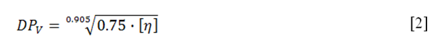Dissolution in a CED solution has been reported to give further depolymerization of TEMPO-oxidized pulps (Kitaoka et al. 1999; da Silva Perez et al. 2003), e.g., a sample of TEMPO-oxidized pulp dissolved in a CED solution was reported to have a DP of about 250 instead of about 500 when the same pulp was dissolved in SO2-diethylamine-dimethylsulfoxide. Thus, this method may overestimate the difference in the DPV between unoxidized pulps and oxidized pulps, but it can be used for a relative compar-ison.

The water retention value (WRV) was analyzed according to SCAN-C 62:00. The analysis deviated from the standard because 0.5 g of pulp was used (instead of 1 g of pulp) and no duplicates were made.

Free swelling capacity (FSC) and centrifuge retention capacity (CRC) were measured according to Edana 440.1-99 and Edana 441.1-99. FSC analysis involves soaking samples in a saline solution for specified intervals and measuring their liquid uptake (by weight) after 1, 5, and 30 min of absorption time. CRC is the amount of liquid retained in a material after centrifugation. The methods deviated from the standard in two ways: the bags were allowed to drip for 2 min before FSC weight measurements, and the sample amount was 25% of a test pad (0.03 to 0.25 g). The results from these measurements are reported as mean values based on four samples.

THEORY

Modeling of TEMPO Oxidation Kinetics

In the modeling of the reaction kinetics, four models were evaluated:

• Model 1: Shrinking core: The diffusion of hypochlorite ions was the rate-determining step.
• Model 2: Shrinking core: Both diffusion and reaction involving hypochlorite ions were the rate-determining steps.
• Model 3: Homogeneous reaction model where the kinetics was described with a first-order reaction with respect to the concentration of hypochlorite ions.
• Model 4: Homogeneous reaction model where the kinetics was described with a first-order reaction with respect to the concentration of primary hydroxyl groups on cellulose.

In the two shrinking core models, it was assumed that the reactions occur only on the surface of the non-oxidized material in the fibre wall at radius ri (Fig. 1). In this case, the reaction occurs initially only on the outer surface of the fibre wall. As the reaction proceeds, the radius of the non-oxidized core shrinks with time as the reaction proceeds inwards.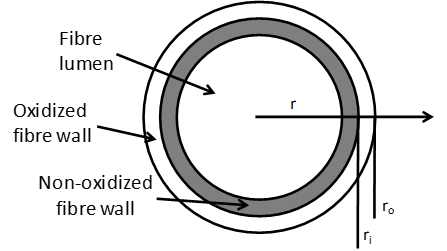Fig. 1. Cross-section of a pulp fibre with radius ro

All four models have their origin in the general transport equation, Eq. . Cylindrical coordinates have been chosen because pulp fibres have a shape that can be approximated with a hollow cylinder of infinite length.The convective term in all the models was cancelled due to the assumption that no flow occurred in the fibre wall. In Model 1, the reaction term was also cancelled based on the assumption that the diffusion of hypochlorite ions was slower than the reaction, i.e., the reaction rate was determined by the rate of diffusion.

In Models 3 and 4, the diffusion term was cancelled, thus neglecting the mass transfer resistance in the fibre wall. Detailed descriptions of Models 1 through 4 are found in the Appendix.

Reactions

TEMPO is present as three different species in the reaction system (Fig. 2), i.e., the nitrosium ion (A), the nitroxyl radical (B), and the hydroxyl amine (C).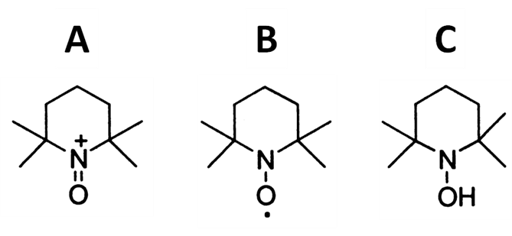Fig. 2. The three co-existing species of TEMPO in the reaction system: A = nitrosium ion, B = nitroxyl radical, and C = hydroxyl amine

The reactions involved in TEMPO oxidation of cellulose have been described in de Nooy et al. (1995), Bragd et al. (2000), and Sun et al. (2005). The nitrosium ion is involved in the reactions where primary hydroxyl groups on the cellulose fibres are oxidized to carboxylic acids via aldehyde intermediates. In these reactions, nitrosium ions are reduced to hydroxyl amines. The formed carboxylic acids are ionised because the reaction is performed at alkaline pH.The hydroxyl amines are regenerated back into nitrosium ions in reactions involving the nitroxyl radical (B) and the secondary oxidant (BrO).The secondary oxidant is regenerated by the primary oxidant (ClO-).The overall reaction of the oxidation of primary hydroxyl groups can thus be written as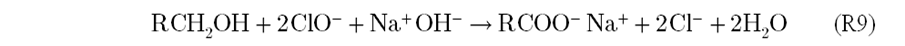RESULTS AND DISCUSSION

Pulp Properties

TEMPO oxidation treatment was performed on softwood kraft pulp at three different dosages of hypochlorite ions and four different temperatures. Table 1 shows that the oxidation rate increased substantially as the reaction temperature increased. At the same dosage of sodium hypochlorite, the differences in the amount of carboxylate groups in the oxidized pulps were also small (less than 15%). There seems to be a maximum in the amount of carboxylate groups when using a reaction temperature of about 30 to 40˚C. However, in some cases, these differences were not large enough to be significant. The selectivity of forming carboxylate groups was about 70 to 75% for the lowest dosage of primary oxidant and 50 to 60% for the two highest dosages of primary oxidant.

The harshest conditions resulted in a 5 to 15% reduction in pulp yield, while less harsh conditions increased the pulp yield slightly. The yield reported here is the gravimetric yield, and yields higher than 100% are feasible for oxidized pulps, mainly due to the introduction of new carboxylate groups with a sodium counter-ion. These groups contributed to the yield, which was calculated to result in a 4 to 6% increase in pulp yield. The yield reduction, at harsh conditions, was probably the result of the dissolution of carbohydrates during the oxidation and washing of the pulp.

The intrinsic viscosity measurements showed that all the oxidized pulp samples had a low viscosimetric degree of polymerization (DPV), in agreement with Kitaoka et al. (1999), compared to the non-oxidized reference pulp (DPV 1250). The trends seen in the data for DPV are to be expected because a higher temperature and a higher dosage of sodium hypochlorite should result in more extensive degradation of the cellulose polymers. This behavior may, at least partly, be explained by β-elimination reactions that occur during TEMPO oxidation at alkaline conditions (de Nooy et al. 1996; Fujisawa et al. 2010). Some additional degradation in DPV had probably occurred during the analytical procedure, see experimental section.

The WRV analysis showed a distinct increase in WRV, due to the oxidation treatment, and WRV was further increased by an increase in the dosage of the primary oxidant. This finding suggests that the TEMPO oxidation treatment increases the swelling of the fibre wall. An increase in fibre swelling was expected due to the higher amount of carboxylate groups.

Table 1. Experimental Conditions and Properties of the Pulps after TEMPO Oxidation Treatment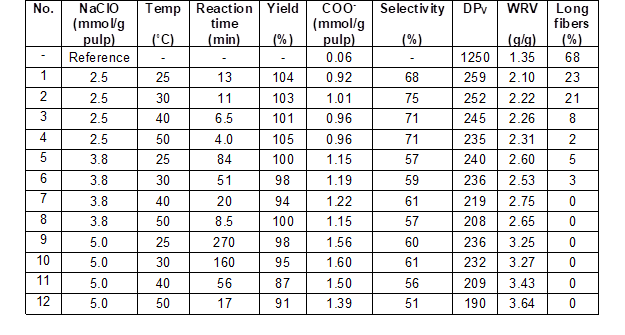Disintegration of TEMPO-oxidized Pulp Fibres

A high-shear dispersing unit was used to disintegrate the TEMPO-oxidized pulp fibres into MFC. In Fig. 3, the fibre length after 3 min of treatment (per g of pulp) is plotted versus the dosage of hypochlorite ions. The fibre length was reduced to a larger extent if the pulp had been oxidized at harsh conditions (high reaction temperature and high dosage of sodium hypochlorite). Fibre length was strongly reduced after only 1 min of treatment (per g of pulp), especially in the samples oxidized at harsh conditions. At high reaction temperatures, the pulp fibres were more chemically degraded, as indicated by a lower pulp yield and DPV, and could therefore be disintegrated with low energy demand. In a recent study (Brodin and Theliander 2012), where the same equipment was used to disintegrate softwood kraft pulp fibres, it was found that non-oxidized fibres were much more difficult to disintegrate than oxidized fibres. After 24 min of treatment (per g of pulp), the non-oxidized pulp had a fibre length of 0.5 mm. Consequently, TEMPO oxidation at harsh conditions makes it possible to disintegrate pulp fibres into MFC with a substantially lower energy demand than for non-oxidized pulp.Fig. 3. Average fibre length (Kajaani FS-300) after 3 min of disintegration treatment plotted versus the dosage of sodium hypochlorite at four oxidation temperatures

Mechanical fractionation of long fibers showed the same trend as fibre analysis (Table 1 and Fig. 3). However, the fibre analyser was also able to detect differences between the samples treated at the harshest conditions during the oxidation treatment, while mechanical fractionation did not retain any long fibres in these samples. In Fig. 4, the particle size distributions in the disintegrated pulp samples (after mechanical fractionation) show that the size distributions became narrower and the particles became smaller when a higher reaction temperature was applied and/or when the dosage of sodium hypochlorite was higher. As the dosage of sodium hypochlorite increased, the disintegration of the fibres became faster and the size distribution shifted towards smaller particles. At the lowest dosage of sodium hypochlorite, it can clearly be seen that a fraction of relatively large pulp fragments (~500 μm) had not been disintegrated (Fig. 4). Furthermore, Fig. 4 shows that the oxidation temperature had a major influence on the disintegration rate at the highest dosage of sodium hypochlorite. This finding demonstrates the powerful effect of chemical degradation on the ease of disintegration.Fig. 4. The frequency of particle size (Mastersizer 2000) is plotted versus particle size (logarithic scale) after 3 min of treatment per gram of pulp. Left: Three different dosages of sodium hypochlorite for pulps oxidized at 50 ˚C. Right: Dosage of sodium hypochlorite was 5.0 mmol/g pulp for all four temperatures.

Absorption and Retention in Freeze-Dried Samples

Figure 5 shows that absorbent materials with good absorption and retention capacities could be produced at all the oxidation conditions evaluated in this study. It was also found that the dosage of primary oxidant had a slightly larger impact on the absorption and retention capacities than reaction temperature. The differences in chemical and physical properties caused by the variations in reaction temperature did not result in any major differences in absorption properties. In agreement with the results presented in Brodin and Theliander (2012), the absorbent materials of this study had a rapid absorption rate, and a higher dosage of primary oxidant yielded absorbent materials with improved retention capacities.Fig. 5. Absorption capacity (FSC) in 0.9 weight-% NaCl solution after 30 min of absorption time and retention capacity (CRC) plotted versus dosage of primary oxidant. The x-axis starts at 2 mmol NaClO/g pulp.

Oxidation Kinetics

In this part of the kinetics study, the decrease in hypochlorite ion concentration due to oxidation reactions was estimated based on the consumption of sodium hydroxide (Fig. 6).Fig. 6. Consumption of hypochlorite ions (50% of total consumption) and sodium hydroxide plotted versus reaction time for oxidation at 30 ˚C and 50 ˚C. Hypochlorite consumption was divided by two because two hypochlorite ions are consumed to oxidize one hydroxyl group to a carboxylic acid.

In Fig. 6, sodium hydroxide and half of the hypochlorite consumption (because two hypochlorite ions are consumed to form one carboxylate group) are shown at two reaction temperatures. The consumption of hypochlorite ions and hydroxide ions correlated well, with a ratio between hypochlorite ions and sodium hydroxide of 2:1. In the initial period of the reaction, the consumption of hypochlorite ions was more rapid than the consumption of sodium hydroxide, which to a large extent is explained by the build-up of the intermediate reaction product (aldehyde groups) (e.g. Saito and Isogai 2004). After this build-up phase, the amount of aldehyde groups on the pulp fibres is expected to be relatively stable due to the fast conversion of aldehyde groups to carboxylate groups (de Nooy et al. 1995).

The results presented in Table 1 show that not all the consumption of hypochlorite ions resulted in carboxylate groups in the final pulp (after oxidation and washing). The amount of carboxylate groups corresponded to about 75% of the hypochlorite consumption at the lowest dosage of primary oxidant and about 50% of the hypochlorite consumption at the highest dosage of primary oxidant. This can in part be explained by the material losses that occurred during the oxidation of the pulp and that had been quantified by determining the pulp yield. The material losses can be explained by oxidized polymers of cellulose or hemicellulose that had been made soluble by oxidation reactions and then transported to the bulk liquid. It is possible that these carbohydrates had been further oxidized in the bulk liquid, without any contribution to the amount of carboxylate groups in the pulp, but nevertheless contributed to the consumption of hypochlorite and hydroxide ions. In these kinetics studies, it was assumed that all reactions involving the substrate, i.e., R1 through R3, had an equal reaction rate.

Kinetics Models 1 through 3

In a first attempt to model the kinetics of the reaction, Models 1 through 3 (see Appendix) were developed to determine if the diffusion of hypochlorite ions limits the rate of the reaction. As shown in Fig. 7, all three models fitted well to the experimental data.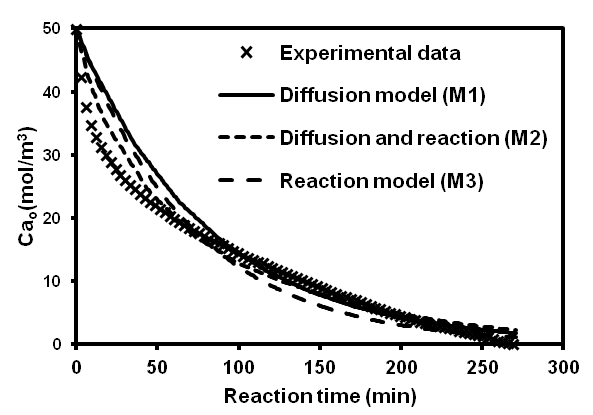Fig. 7. Experimental data on hypochlorite ion concentration calculated from data on hydroxide ion consumption is plotted versus the reaction time together with simulation data from the three kinetics models. The experimental conditions for this data are at 25 ˚C oxidation temperature and 5.0 mmol NaClO/g pulp.

The values of the coefficients for all reaction conditions, in Table 2, show that both the diffusion coefficients and the rate constants have values of reasonable size. However, in a comparison of the diffusion coefficients in Model 1 (De1), it was found that the coefficients had lower values at high dosages than at low dosages of sodium hypochlorite, which was not expected for a diffusion-controlled system. The rate constants (k3) show the same trend, where a higher dosage of sodium hypochlorite results in a lower value for the rate constant.

Table 2. Data on Effective Diffusivity Coefficients (Models 1 And 2) and Rate Constants (Models 2 and 3) from the Model Fitting in MATLAB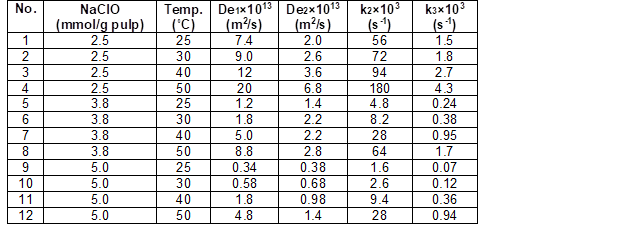In addition, Fig. 8 shows that the concentration of primary oxidant did not have any significant influence on the rate of the reaction, at least not during the first minutes of the reaction. The same trend was also observed at the other three reaction temperatures, showing that the initial reaction rate was not influenced by the concentration of hypochlorite ions.Fig. 8. Consumption of NaOH plotted versus reaction time for three dosages of primary oxidant at a reaction temperature of 50 ˚C

These findings together show that the reaction rate in TEMPO oxidation of kraft pulp fibres was not limited by the dosage of hypochlorite ions at the conditions used. Consequently, the diffusion of hypochlorite ions from the bulk liquid to the reaction sites in the fibre wall was sufficiently fast at the conditions investigated.

Oxidation Kinetics – Model 4

First-order kinetics with respect to the concentration of primary hydroxyl groups on cellulose was studied by plotting ln(Cprim-OHversus the reaction time. The rate constant (the slope of the curve) decreased during the reaction time (Fig. 9). Three periods were found: an initial period with a rapid reaction rate (high value of rate constant), an intermediate period with a gradual decrease rate (not following first-order kinetics), and an end period with a slow reaction rate (low value of the rate constant). Each of these periods represented about 1/3 of the total decrease in the concentration of primary hydroxyl groups. It appears that there were at least two rate-determining steps, and that the rate-limiting step changed from the initial period to the end period.Fig. 9. Linear fitting of the initial and the end phase of the reaction for reaction temperatures from 25 ˚C to 50 ˚C

The rate constant during the more rapid initial reaction period was about four to five times the value of the rate constant of the slower end phase (Table 3). The values of the rate constants, as shown in the table, show that the reaction rate was strongly influenced by the reaction temperature. An increase in temperature from 25 ˚C to 50 ˚C resulted in an increase in rate constants by a factor of about six.

Table 3. Rate Constants Based on the Concentration of Primary Hydroxyl Groups at Different Conditions. [NaClO = 5.0 mmol/g pulp; pulp consistency = 1%]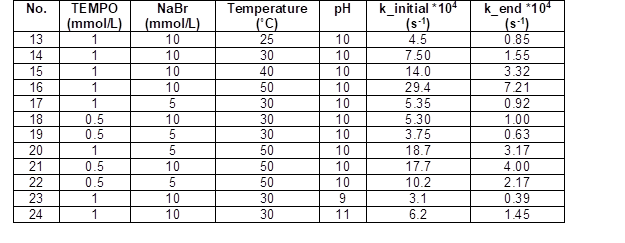Activation Energy

The activation energy was determined by plotting the rate constants in the range from 25 ˚C to 50 ˚C (Fig. 10). The activation energy of the TEMPO oxidation of kraft pulp was 58.4 kJ/mol for the initial phase and 66.9 kJ/mol for the end phase. These values for the activation energy are similar to values reported for TEMPO oxidation of various substrates, e.g., 58 kJ/mol for α-D-glucopyranoside (de Nooy et al. 1995), 66.2 kJ/mol for rayon (Sun et al. 2005), and 56.7 kJ/mol for cotton linter (Dai et al. 2011).Fig. 10. Arrhenius plot of TEMPO oxidation of softwood kraft pulp fibres in the range of 25 ˚C to 50 ˚C

Influence of Catalytic Components and pH

Figure 11 shows that the reaction rate of the TEMPO/NaBr/NaClO system was not affected by the concentration of hypochlorite ions. When performing the reaction without NaBr, the reaction rate became much slower; to achieve a more realistic reaction rate, the TEMPO concentration was increased by a factor of five. At this TEMPO concentration and in the absence of bromide, the rate constant increased from 0.58×10-4 s-1 to 1.42×10-4 s-1 by doubling the dosage of hypochlorite ions. Thus, in the presence of bromide, the generation of the nitrosium ion was sufficiently fast, but in the absence of bromide and with a 500% increase in TEMPO concentration, the regeneration of TEMPO by hypochlorite ions became rate limiting. This result is in contrast to the findings of Bragd et al. (2000), who found that in bromide-free oxidation of α-D-glucopyranoside, no change in rate was observed when changing the concentration of hypochlorite ions.Fig. 11. NaOH consumption during a TEMPO oxidation with NaBr (left) and a TEMPO oxidation without NaBr (right) using two different dosages of primary oxidant

Table 3 shows that the reaction rate was affected by the concentrations of both TEMPO and NaBr at reaction temperatures of 30 ˚C and 50 ˚C. An increase or decrease in either TEMPO or NaBr had more or less the same effect at the investigated conditions. In this case, the concentration of the active TEMPO compound, i.e., the nitrosium ion, was most likely the same because basically the same rate constant was obtained. The results suggest that both the concentrations of TEMPO and NaBr should be included in a model to describe the kinetics of the reaction. Consequently, it would be advantageous if the concentrations of hypobromite and nitrosium ions could be measured during the course of the reaction.

The reaction pH also influenced the rate of the reaction, as shown in Table 3 and in agreement with Bragd et al. (2000). The highest rate was obtained at about pH 10 for TEMPO oxidation in the presence of NaBr. This optimum in reaction rate shows that the hydroxide ion concentration is important, but because it is involved in several reactions (R1 through R6), this finding gives no additional information regarding the rate-determining step. At a lower pH, a larger fraction of the hypochlorite and hypobromite will be protonated. On the other hand, a high pH will also increase the degradation of the pulp through alkaline hydrolysis (Fujisawa et al. 2010), and at pH above 9, it has been reported that nitrosium ions react with hydroxide ions to form nitroxyl radicals and hydrogen peroxide (Endo et al. 1984).

To sum up, all four models investigated in this study could be fitted to the experimental data. However, none of the models could describe all the mechanisms involved. The results strongly indicate that more than one step limits the reaction rate, but it still remains to be explained which reactions are responsible for which phases. It cannot be excluded that more than one reaction should be included, as it was found that the concentrations of both NaBr and TEMPO have a strong influence on the reaction rate. The mass transfer of hypochlorite ions was sufficiently fast to not affect the rate of the reaction, but it is possible that the mass transfer of any of the catalytic compounds limited the reaction rate in the later phases of the reaction. Methods for measuring the concentration of the active catalytic compounds during the reaction are needed, as experimental data for hydroxide ions together with hypochlorite concentration do not explain the kinetics of all the reactions involved.

CONCLUSIONS

1. TEMPO oxidation of pulp fibres at a high oxidation temperature reduced the reaction time compared to TEMPO oxidation at moderate temperatures, but the higher oxidation temperature also caused more extensive degradation of the carbohydrate polymers in the pulp sample. Only minor effects on the amount of carboxylate groups were detected by varying the reaction temperature in the range between 25 ˚C and 50 ˚C.
2. Disintegration of the oxidized pulp fibres showed that the use of harsh oxidation conditions (high temperature and high dosage of sodium hypochlorite) enabled a more rapid disintegration, thus reducing the demand for energy in this operation.
3. The capacities ranged from 40 and 80 g/g for absorption and 9 and 14 g/g for liquid retention. The reaction temperature had minor to no influence on the absorption and retention capacities of the freeze-dried absorbent material.
4. Four kinetic models were fitted to experimental data, but none of the models could describe all the mechanisms involved in the TEMPO oxidation of pulp fibres. Nevertheless, it was found that the diffusion of hypochlorite ions in the fibre wall was sufficiently fast in the TEMPO/NaBr/NaClO system so as to not limit the rate of the reaction. Modelling with a homogenous first-order reaction with respect to the concentration of primary hydroxyl groups showed that the reaction shifts between two rate liming steps during the reaction: one in the rapid initial phase and one in the end phase of the reaction. However, the rate-limiting steps behind the different phases of the reaction still remain to be explained.
5. The reaction rate was strongly influenced by the reaction temperature as well as the concentration of the catalytic components (NaBr and TEMPO). The reaction rate was also found to be the highest at a pH level of around 10, in agreement with earlier findings.

ACKNOWLEDGMENTS

The foundation “Södra skogsägarnas stiftelse för forskning, utveckling och utbildning”, SCA Hygiene Products AB, and Vinnova are gratefully acknowledged for financial support. This study was conducted within the WooDi Project, which is a sub-project in “Vinnovas branschforskningsprogram för skogs-och träindustrin” (Vinnovas Sectoral R&D Programme for the Forest-based Industry).

REFERENCES CITED

Bragd, P., Besemer, A., and van Bekkum, H. (2000). “Bromide-free TEMPO-mediated oxidation of primary alcohol groups in starch and methyl α-D-glucopyranoside,” Carbohydr. Res.328(3), 355-363.

Bragd, P., van Bekkum, H., and Besemer, A. (2004). “TEMPO-mediated oxidation of polysaccharides: Survey of methods and applications,” Top. Catal. 27(1-4), 49-66.

Brodin, F., and Theliander, H. (2012). “Absorbent materials based on kraft pulp: Preparation and material characterization,” BioResources 7(2), 1666-1683.

da Silva Perez, D., Montanari, S., and Vignon, M. (2003). “TEMPO-mediated oxidation of cellulose III,” Biomacromolecules 4(5), 1417-1425.

Dai, L., Dai, H., Yuan, Y., Sun, X., and Zhu, Z. (2011). “Effect of TEMPO oxidation system on kinetic constants of cotton fibres,” BioResources 6(3), 2619-2631.

Dang, Z., Zhang, J., and Ragauskas, A. (2007). “Characterizing TEMPO-mediated oxidation of ECF bleached softwood kraft pulps,” Carbohydr. Polym. 70(3), 310-317.

de Nooy, A., Besemer, A., and van Bekkum, H. (1995). “Selective oxidation of primary alcohols mediated by nitroxyl radicals in aqueous solution. Kinetics and mechanism,” Tetrahedron51(29), 8023-8032.

de Nooy, A., Besemer, A., van Bekkum, H., van Dijk, J., and Smith, J. (1996). “TEMPO-mediated oxidation of pullan and influence of ionic strength and linear charge density on the dimensions of the obtained polyelectrolyte chains,” Macromolecules 29(20), 6541-6547.

Endo, T., Miyazawa, T., Shiihashi, S., and Okawara, M. (1984). “Oxidation of hydroxide ion by immonium oxide,” J. Am. Chem. Soc. 106(13), 3877-3878.

Fujisawa, S., Isogai, T., and Isogai, A. (2010). “Temperature and pH stability of celluronic acid,” Cellulose 17(3), 607-615.

Isogai, A., and Kato, Y. (1998). “Preparation of polyuronic acid from cellulose by TEMPO-mediated oxidation,” Cellulose 5(3), 153-164.

Kitaoka, T., Isogai, A., and Onabe, F. (1999). “Chemical modification of pulp fibres by TEMPO mediated oxidation,” Nord. Pulp Pap. Res. J. 14(4), 279-284.

Saito, T., and Isogai, A. (2004). “TEMPO-mediated oxidation of native cellulose. The effect of oxidation conditions on chemical and crystal structures of the water-insoluble fractions,” Biomacromolecules 5(5), 1983-1989.

Saito, T., Nishiyama, Y., Putaux, J.-L., Vignon, M., and Isogai, A. (2006). “Homogeneous suspensions of individualized microfibrils from TEMPO-catalyzed oxidation of native cellulose,” Biomacromolecules 7(6), 1687-1691.

Saito, T., Hirota, M., Tamura, N., Kimura, S., Fukuzumi, H., Heux, L., and Isogai, A. (2009). “Individualization of nano-sized plant cellulose fibrils by direct surface carboxylation using TEMPO catalyst under neutral conditions,” Biomacromolecules 10(7), 1992-1996.

Sun, B., Gu, C., Ma, J., and Liang, B. (2005). “Kinetic study on TEMPO-mediated selective oxidation of regenerated cellulose,” Cellulose 12(1), 59-66.

Article submitted: May 16, 2013; Peer review completed: September 16, 2013; Revised version received and accepted: September 25, 2013; Published: October 1, 2013.

APPENDIX

In this appendix, detailed descriptions of the four kinetics models are presented.

The experimental data (Models 1 through 3)

Parameters related to hypochlorite ion consumption were calculated from the experimental data on sodium hydroxide consumption in the oxidation experiments. It was assumed that the consumption of hypochlorite ions was proportional to the consumption of hydroxide ions. The actual concentration of hypochlorite ions during the reactions was not measured in the experiments in the first part of the kinetic study. However, the TEMPO oxidation system is complex, and many reactions occur in parallel involving both hydroxide ions and hypochlorite ions. For example, in the beginning of the reaction, more aldehyde groups are introduced than carboxylate groups, which will cause a deviation in the relation between hypochlorite concentration and hydroxide ion consumption, as discussed in the section “Results and Discussion.” It has been estimated that the concentration of hypochlorite ions may be overestimated by a maximum between 4% (the highest dosage of NaClO) and 8% (the lowest dosage of NaClO) in the early stage of the reaction, but this error probably decreases as the reaction continues. Minor errors may also come from unselective reactions between hypochlorite or hydroxide ions and the carbohydrates.

Assumptions

A number of assumptions were made in the construction of the two shrinking core models, and many of them originate from shrinking core theory (Models 1 and 2):

• The reactions were assumed to occur in an infinitely small reaction volume in the fibre wall that gradually moves inwards as the reaction proceeds.
• The fibres were assumed to have the shape of hollow cylinders with closed ends with an outer radius of 25 μm and a fibre wall thickness of 3.2 μm.
• The concentration of chemicals inside the fibre lumen was assumed to be zero; thus, the transport of chemicals though pits in the fibre wall was neglected.
• Equi-molar counter-diffusion was assumed for reactants and products in the fibre wall.
• Although the radius of the shrinking core changes with time, the concentration profile of hypochlorite ions in the fibre wall was assumed to be at steady state (quasi-steady state).
• It was assumed that the carboxylate groups were evenly distributed in the fibre wall at the end of the reaction.
• External mass transfer resistance was neglected.

Some assumptions were also made in Model 3:

• All chemicals and substrates were assumed to be evenly distributed in the reaction vessel.
• The reactions with hypochlorite ions were assumed to be of the first order with respect to the hypochlorite ion.
• The last assumption was that all reactions occurred simultaneously and in the same proportions during the reaction time period.

Model 1. Shrinking Core: The Diffusion of Hypochlorite Ions was the Rate-Determining Step

The transport equation in the diffusion model that describes the diffusion of hypochlorite ions into the fibre wall of the pulp fibres is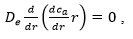[A1]

where De is the effective diffusivity in the porous fibre wall and the subscript a is the notation of hypochlorite ions. The boundary conditions used to solve this equation were

BC1: at r = ro c= cao(t)

BC2: at r = ri(t) ca = 0 ,

where ro and cao are the radius and the concentration at the outer surface of the fibre, respectively, and r(t) is the time-dependent radius at the surface of the shrinking core. The solution to Eq. [A1] is presented below,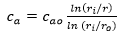[A2]

where ca is the concentration of hypochlorite ions and cao is the time-dependent concentration of hypochlorite ions at the outer fibre surface.

Mass balances of the key components in the system must then be formed to create a mathematical model. The first mass balance correlates with the fibre wall material, including a reaction term for the surface reaction on the surface of the shrinking core (left) and an accumulation term (right) for the decrease in the unreacted fibre wall volume,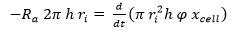[A3]

where Ra is the rate at which carboxylate groups are formed, h is the total length of the fibres, φ is the molar density of primary hydroxyl groups in the cell wall, and xcell is the mean fraction of primary hydroxyls that react with carboxylate groups according to experimental results. The equation for the xcell factor can be found below. Eq. [A3] can be simplified to a differential equation that describes the change in radius of the shrinking core.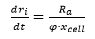[A4]

In a diffusion model without a reaction term, the reaction is considered to be rapid, implying that the overall rate of the oxidation reaction in this model is limited by the diffusion of hypochlorite ions. The molar flux of hypochlorite ions Wa,r in the fibre wall can be expressed by Fick´s First Law: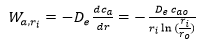[A5]

The shrinking core theory assumes that the reaction occurs at the surface of the non-reacted material core. Therefore, the molar flux at radius ri must be equal to the reaction rate: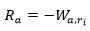[A6]

Consequently, the reaction rate Ra can be expressed by the molar flux of hypochlorite in the fibre wall at radius ri. Therefore, Eq. [A4] can be rewritten as,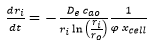[A7]

where the first term describes the molar flux of hypochlorite ions.

The second mass balance treats the consumption of hypochlorite ions in the bulk solution, expressed in molar units,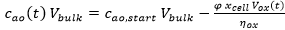[A8]

where cao,start is the initial concentration of hypochlorite ions in the bulk liquid, Vbulk is the volume of bulk liquid, and Vox(t) is the volume of oxidized fibre material at time t.

Model 2. Shrinking Core: Both Diffusion and Reaction Involving Hypochlorite Ions were the Rate-Determining Steps

The transport equation in the two-parameter model that describes the diffusion of hypochlorite ions into the cell wall of pulp fibres combined with a chemical reaction is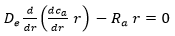, [A9]

where De is the effective diffusivity in the porous cell wall of the fibres and the subscript a is the notation of hypochlorite ions. The reaction Ra is assumed to be a first-order reaction (Ra = kca). The boundary conditions used to solve Eq. [A9] were

BC1: at r = 0 cis finite

BC2: at r = ro ca = cao ,

where ro is the radius at the outer surface of the fibre. The solution of Eq. [A9] is presented below,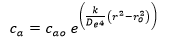[A10]

where ca is the concentration of hypochlorite ions at radius r and cao is the time-dependent concentration of hypochlorite ions at the outer fibre surface.

The first mass balance that treats the unreacted fibre wall material is analogous to Model 1, but due to the different concentration gradient at radius ri (the surface of the shrinking core), the expression of the shrinking core radius in this case becomes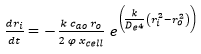, [A11]

where k is the reaction constant for the overall oxidation reaction.

The second mass balance for the disappearance of hypochlorite ions is the same as in Model 1, cf. Eq. [A8].

Model 3. Homogeneous Reaction Model where the Kinetics was Described with a First-Order Reaction with Respect to the Concentration of Hypochlorite Ions

In this model, the overall reaction rate equation is composed of a reaction term and an accumulation term, as shown in Eq. [A12],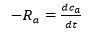[A12]

where the reaction rate Ra can be described as a first-order reaction,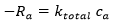[A13]

where ktotal is the total reaction constant of reactions that consume hypochlorite ions and ca is the bulk concentration of hypochlorite ions. The solution to Eq. [A12] is,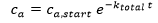[A14]

where ca,start is the initial concentration of hypochlorite ions. Finally, the total reaction constant must be multiplied by the efficiency factor ηox to obtain the reaction rate of reaction R2. The equation for ηox is shown below: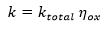[A15]

The xcell factor is calculated in a mole balance according to,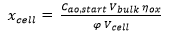[A16]

where Cao,start is the concentration of hypochlorite ions at time zero, Vbulk is the volume of liquid in the reaction, and ηox is the efficiency of hypochlorite ions for generating carboxylate groups. The numerator in Eq. [A16] describes how many hypochlorite ions are consumed in the second oxidation reaction, and the denominator describes the total amount of primary hydroxyl groups in the cell wall of the fibres. The efficiency factor ηox is calculated using the following equation,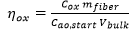[A17]

where Cox is the concentration of carboxylate groups per gram of pulp and mfibre is the weight of fibres used in the reaction. The efficiency describes the fraction of total added hypochlorite ions used in the second oxidation reaction, where carbonyl groups are oxidized to carboxylate groups.

Model 4. Homogeneous Reaction Model where the Kinetics was Described with a First-Order Reaction with Respect to the Concentration of Primary Hydroxyl Groups on Cellulose

In the second part of the kinetic study, a homogeneous reaction model was used according to Bragd et al. (2000). They proposed a kinetic expression for TEMPO oxidation that assumes that the reaction between the primary hydroxyl groups (prim-OH) and the nitrosium ions (TEMPO (A)), Reaction R1, is the rate determining step,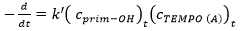[A18]

where k’ is the rate constant. By assuming that the concentration of nitrosium ions is in steady state, the follow expression was obtained,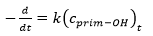[A19]

where k represents the observed reaction rate. The following rate expression is obtained through integration of Equation [A19]: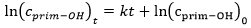[A20]

When plotting ln(cprim-OH)t versus t, the rate constant k is equal to the slope if the plot results in a straight line. The amount of primary hydroxyl groups reacted in the TEMPO oxidation reaction was estimated by the consumption of sodium hydroxide, i.e., one mole of primary hydroxyl group per one mole of sodium hydroxide.

NOMENCLATURE

ca Concentration of hypochlorite ions (mol/m3)

cao Concentration of hypochlorite ions on the outer surface of the fibre wall (mol/m3)

cao,start Starting concentration of hypochlorite ions on the outer surface of the fibre (mol/m3)

cprim-OH Concentration of primary hydroxyl groups (mol/m3)

cTEMPO (A) Concentration of nitrosium ions (mol/m3)

DEffective diffusion coefficient (m2/s)

k Reaction rate constant (s-1)

Reaction rate constant (mol/m3 s-1)

ktotal Total reaction rate constant of all reactions that consumes hypochlorite ions both selective and non-selective reactions. (s-1)

r Radius, i.e., distance from the centre of the fibre (m)

Ra Reaction rate of formation of carboxylate groups (mol/m× s)

ri Radius of the shrinking core in the fibre wall (m)

ro Radius at the outer fibre surface (m)

t Reaction time (s)

Vbulk Volume of bulk liquid (m3)

Vox Volume of fibre wall (m3)

Wa,r Molar flux of hypochlorite ions in the fibre wall (mol/m× s)

xcell Fraction of total primary hydroxyl groups that are oxidized (calculated from experimental data)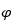Molar density of primary hydroxyl groups in the fibre wall (mol/m3)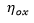Fraction of hypochlorite ions that is consumed in reaction R2 (calculated from experimental data)